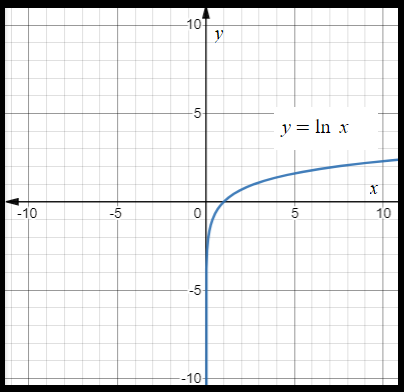1
41
views
61a
Problem

Problem 61a

Chapter 1: Functions and ModelsTextbook ExpertVerified Tutor
18 Oct 2021

Given information

Given equation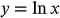shift the graph of the given equation by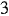units.

Step-by-step explanation

Step 1.

If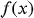is shifted by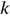units upward, the resultant graph is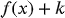.

Draw the graph of.